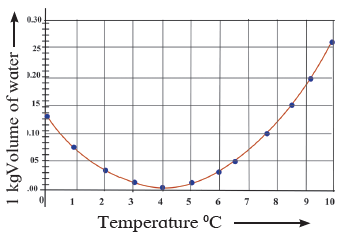### Question 2. Observe the following graph. Considering the change in volume of water as its temperature is raised from 0oC, discuss the difference in the behaviour of water and other substances. What is this behavior of water called?

Question 2.

Observe the following graph. Considering the change in volume of water as its temperature is raised from 0oC, discuss the difference in the behaviour of water and other substances. What is this behavior of water called?The given figure is the graph between the volume and temperature of water.

(i) If the temperature is raised from 0°C, this means heating takes place.

(ii) It has been observed that on heating, it contracts instead of expanding.

(iii) At 4° C, due to contraction, volume decreases.

(iv) If the water is heated further, it expands and its volume increases.

(v) The behaviour of water between its temperature from 4° C, to 0° C, is called anomalous behaviour of water.